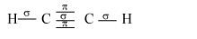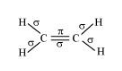# What is the total number of sigma and pi bonds in the following molecules?Question:

What is the total number of sigma and pi bonds in the following molecules?

(a) C2H2

(b) C2H4

Solution:

A single bond is a result of the axial overlap of bonding orbitals. Hence, it contributes a sigma bond. A multiple bond (double or triple bond) is always formed as a result of the sidewise overlap of orbitals. A pi-bond is always present in it. A triple bond is a combination of two pi-bonds and one sigma bond.

Structure of C2H2 can be represented as:Hence, there are three sigma and two pi-bonds in C2H2.

The structure of C2H4 can be represented as:Hence, there are five sigma bonds and one pi-bond in C2H4.